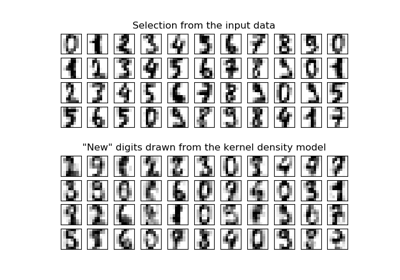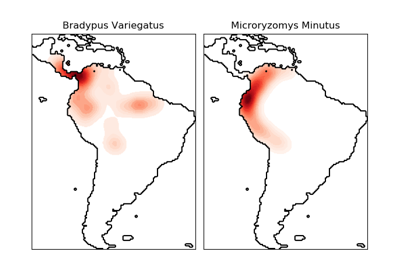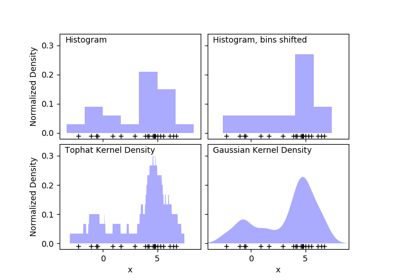# sklearn.neighbors.KernelDensity¶

class sklearn.neighbors.KernelDensity(bandwidth=1.0, algorithm='auto', kernel='gaussian', metric='euclidean', atol=0, rtol=0, breadth_first=True, leaf_size=40, metric_params=None)[source]

Kernel Density Estimation

Read more in the User Guide.

Parameters: bandwidth : float The bandwidth of the kernel. algorithm : string The tree algorithm to use. Valid options are [‘kd_tree’|’ball_tree’|’auto’]. Default is ‘auto’. kernel : string The kernel to use. Valid kernels are [‘gaussian’|’tophat’|’epanechnikov’|’exponential’|’linear’|’cosine’] Default is ‘gaussian’. metric : string The distance metric to use. Note that not all metrics are valid with all algorithms. Refer to the documentation of BallTree and KDTree for a description of available algorithms. Note that the normalization of the density output is correct only for the Euclidean distance metric. Default is ‘euclidean’. atol : float The desired absolute tolerance of the result. A larger tolerance will generally lead to faster execution. Default is 0. rtol : float The desired relative tolerance of the result. A larger tolerance will generally lead to faster execution. Default is 1E-8. breadth_first : boolean If true (default), use a breadth-first approach to the problem. Otherwise use a depth-first approach. leaf_size : int Specify the leaf size of the underlying tree. See BallTree or KDTree for details. Default is 40. metric_params : dict Additional parameters to be passed to the tree for use with the metric. For more information, see the documentation of BallTree or KDTree.

Methods

 fit(X[, y, sample_weight]) Fit the Kernel Density model on the data. get_params([deep]) Get parameters for this estimator. sample([n_samples, random_state]) Generate random samples from the model. score(X[, y]) Compute the total log probability density under the model. score_samples(X) Evaluate the density model on the data. set_params(**params) Set the parameters of this estimator.
__init__(bandwidth=1.0, algorithm='auto', kernel='gaussian', metric='euclidean', atol=0, rtol=0, breadth_first=True, leaf_size=40, metric_params=None)[source]
fit(X, y=None, sample_weight=None)[source]

Fit the Kernel Density model on the data.

Parameters: X : array_like, shape (n_samples, n_features) List of n_features-dimensional data points. Each row corresponds to a single data point. sample_weight : array_like, shape (n_samples,), optional List of sample weights attached to the data X.
get_params(deep=True)[source]

Get parameters for this estimator.

Parameters: deep : boolean, optional If True, will return the parameters for this estimator and contained subobjects that are estimators. params : mapping of string to any Parameter names mapped to their values.
sample(n_samples=1, random_state=None)[source]

Generate random samples from the model.

Currently, this is implemented only for gaussian and tophat kernels.

Parameters: n_samples : int, optional Number of samples to generate. Defaults to 1. random_state : int, RandomState instance or None. default to None If int, random_state is the seed used by the random number generator; If RandomState instance, random_state is the random number generator; If None, the random number generator is the RandomState instance used by np.random. X : array_like, shape (n_samples, n_features) List of samples.
score(X, y=None)[source]

Compute the total log probability density under the model.

Parameters: X : array_like, shape (n_samples, n_features) List of n_features-dimensional data points. Each row corresponds to a single data point. logprob : float Total log-likelihood of the data in X. This is normalized to be a probability density, so the value will be low for high-dimensional data.
score_samples(X)[source]

Evaluate the density model on the data.

Parameters: X : array_like, shape (n_samples, n_features) An array of points to query. Last dimension should match dimension of training data (n_features). density : ndarray, shape (n_samples,) The array of log(density) evaluations. These are normalized to be probability densities, so values will be low for high-dimensional data.
set_params(**params)[source]

Set the parameters of this estimator.

The method works on simple estimators as well as on nested objects (such as pipelines). The latter have parameters of the form <component>__<parameter> so that it’s possible to update each component of a nested object.

Returns: self

## Examples using sklearn.neighbors.KernelDensity¶Kernel Density EstimationKernel Density Estimate of Species DistributionsSimple 1D Kernel Density Estimation